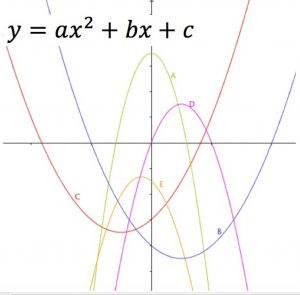The diagram shows the graphs of five quadratic functions or parabolas.

How do the points where the graph cuts the x axis relate to the  axis of symmetry of the graph and the solutions of the quadratic equation $ax^2 + bx + c = 0$?

Match the graphs in the diagram to the following descriptions and give reasons for your decisions.

1. $y = ax^2 + bx + c$ if a > 0, b > 0 and c < 0.
2. $y = ax^2 + bx + c$ if a < 0, b =  0 and c > 0.
3. $y = ax^2 + bx + c$ if a < 0, b <  0 and $b^2 - 4ac < 0$.
4. $y = a(x+p)^2+q$ if p < 0, q < 0 and the x-intercepts have different signs.
5. $y = a(x+p)^2+q$ if a < 0, p < 0, q > 0 and one root is zero.
Tagged with: